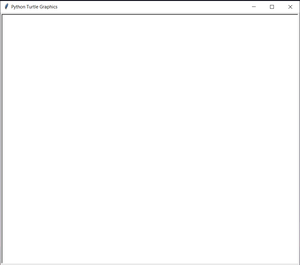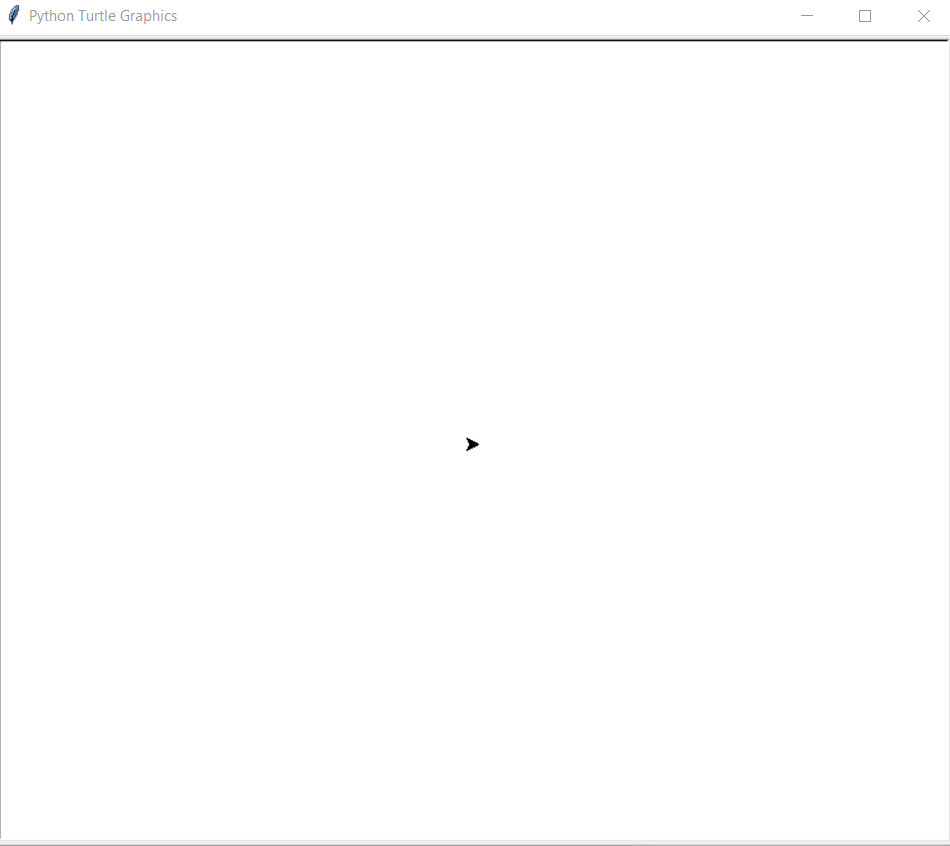# Python – Draw Star Using Turtle Graphics

• Difficulty Level : Medium
• Last Updated : 16 Oct, 2020

In this article, we will learn how to make a Star using Turtle Graphics in Python. For that let’s first know what is Turtle Graphics.

### Turtle graphics

Turtle is a Python feature like a drawing board, which let us command a turtle to draw all over it! We can use many turtle functions which can move the turtle around. Turtle comes into the turtle library. The turtle module can be used in both object-oriented and procedure-oriented ways.

Some commonly used methods are:

• forward(length): moves the pen in the forward direction by x unit.
• backward(length): moves the pen in the backward direction by x unit.
• right(angle): rotate the pen in the clockwise direction by an angle x.
• left(angle): rotate the pen in the anticlockwise direction by an angle x.
• penup(): stop drawing of the turtle pen.
• pendown(): start drawing of the turtle pen.

### Approach

• First import turtle module in the idle or editor you are using.
```import turtle
```
• Get a screen board on which turtle will draw.
```ws=turtle.Screen()
```

A screen like this will appear:-• Define an instance for turtle.
• For a drawing, a Star executes a loop 5 times.
• In every iteration move the turtle 100 units forward and move it right 144 degrees.
• This will make up an angle 36 degrees inside a star.
• 5 iterations will make up a Star perfectly.

Below is the python implementation of the above approach.

First way :

## Python3

 `# import for turtle` `import` `turtle`   `# Starting a Working Screen` `ws ``=` `turtle.Screen()`   `# initializing a turtle instance` `geekyTurtle ``=` `turtle.Turtle()`   `# executing loop 5 times for a star` `for` `i ``in` `range``(``5``):`   `        ``# moving turtle 100 units forward` `        ``geekyTurtle.forward(``100``)`   `        ``# rotating turtle 144 degree right` `        ``geekyTurtle.right(``144``)`

### Output:Turtle Making A Star

Alternate Approach:

## Python3

 `#import turtle` `import` `turtle`   `# set screen` `Screen ``=` `turtle.Turtle()`   `# decide colors` `cir``=` `[``'red'``,``'green'``,``'blue'``,``'yellow'``,``'purple'``]`   `# decide pensize` `turtle.pensize(``4``)`   `# Draw star pattern` `turtle.penup()` `turtle.setpos(``-``90``,``30``)` `turtle.pendown()` `for` `i ``in` `range``(``5``):` `    ``turtle.pencolor(cir[i])` `    ``turtle.forward(``200``)` `    ``turtle.right(``144``)`   `turtle.penup()` `turtle.setpos(``80``,``-``140``)` `turtle.pendown()`   `# choose pen color` `turtle.pencolor(``"Black"``)` `turtle.done()`

Output:-

My Personal Notes arrow_drop_up
Recommended Articles
Page :